# R S Aggarwal Solutions for Class 10 Maths Chapter 10 Trigonometry Ratios

R S Aggarwal Class 10 Solutions for Chapter 10 Trigonometry Ratios are available here. Trigonometry Ratios is composed of only one exercise which includes concepts such as trigonometric ratios of an acute angle of a right triangle, reciprocal relations, T-ratios of an angle, quotient relation of T-ratios and squared relation. All textbook questions are solved by subject experts at BYJU’S based on CBSE Syllabus. Students can avail the R S Aggarwal Solutions for Chapter 10 now and clear their doubts on the chapter.

## Exercise 10 Page No: 539

Question 1:
Solution:

Given function: sin θ = √3/2

Let us first draw a right ∆ABC, ∠B = 90 degrees and ∠A = 𝜃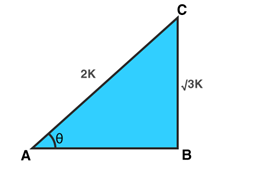(where k is a positive)

We know that sin 𝜃 = BC/AC = (Perpendicular)/Hypotenuse = √3/2

By Pythagoras Theorem:

AC2 = AB2 + BC2

Or AB2 = AC2 – BC2 = 4k2 – 3k2 = k2

AB = k

Find other T-rations using their definitions:

Cos = AB/AC = 1/2

Tan 𝜃 = BC/𝐴𝐵 = √3

𝑐𝑜𝑠𝑒𝑐 𝜃 = 1/sin𝜃 = 2/√3

sec 𝜃 = 1/cos 𝜃 = 2

cot 𝜃 = 1/tan 𝜃 = 1/√3

Question 2:
Solution:

Given function: cos θ = 7/25

Draw a right ∆ABC, ∠B = 90 degrees and ∠A = θ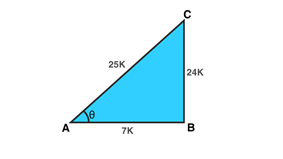(where k is a positive)

We know that cos θ = AB/AC = Base/Hypotenuse = 7/25

By Pythagoras Theorem:

AC2 = AB2 + BC2

Or BC2 = AC2 – AB2 = 625k2 – 49k2 = 576k2

AB = 24k

Find other T-rations using their definitions:

Sin 𝜃 = BC/AC = 24/25

Tan 𝜃 = BC/𝐴𝐵 = 24/7

𝑐𝑜𝑠𝑒𝑐 𝜃 = 1/sin𝜃 = 25/24

sec 𝜃 = 1/cos 𝜃 = 25/7

cot 𝜃 = 1/tan 𝜃 = 7/24

Question 3:
Solution:

Given function: tan θ=15/8

Draw a right ∆ABC, ∠B = 90 degrees and ∠A = θ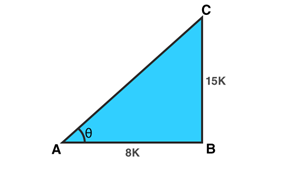We know that tan θ = BC/AB = perpendicular/base = 15/8

(where k is a positive)

By Pythagoras Theorem:

AC2 = AB2 + BC2

= 64k2 + 225k2

= 289k2

AC = 17k

Find other T-rations using their definitions:

Sin 𝜃 = BC/AC = 15/17

cos 𝜃 = AB/AC = 8/17

𝑐𝑜𝑠𝑒𝑐 𝜃 = 1/sin𝜃 = 17/15

sec 𝜃 = 1/cos 𝜃 = 17/8

cot 𝜃 = 1/tan 𝜃 = 8/15

Question 4:
Solution:

Given function: cot θ= 2

Draw a right ∆ABC, ∠C = 90 degrees and ∠A = θ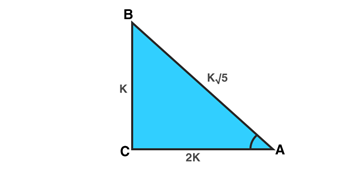We know that cot θ = AC/BC = base/perpendicular = 2/1

(where k is a positive)

By Pythagoras Theorem:

AB2 = BC2 + AC2

= k2 + 4k2

= 5k2

AB = k√5

Find other T-rations using their definitions:

Sin 𝜃 = BC/AB = k/(k√5) = 1/√5

cos 𝜃 = AC/AB = (2k)/(k√5) = 2/√5

tan θ = BC/AC = sinθ /cosθ = k/(2k) = 1/2

𝑐𝑜𝑠𝑒𝑐 𝜃 = 1/sin𝜃 = √5

sec 𝜃 = 1/cos 𝜃 = √5/2

Question 5:
Solution:

Given function: cosec θ = √10

Draw a right ∆ABC, ∠C = 90 degrees and ∠A = θ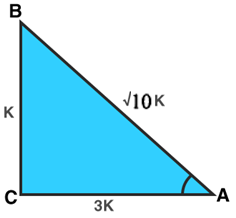We know that, cosecθ = AB/BC = hypotenuse/perpendicular = (k√10)/k

(where k is a positive)

By Pythagoras Theorem:

AB2 = AC2 + BC2

AB2 = AC2 – BC2

= 10k2 – k2

= 9k2

AC = 3k

Find other T-rations using their definitions:

Sin 𝜃 = BC/AB = 1/√10

cos 𝜃 = AC/AB = (3k)/(k√10) = 3/√10

tan θ = BC/AC = sinθ /cosθ = 1/3

secθ = AB/AC = 1/cosθ = √10/3

cotθ = AC/BC = 1/tanθ = 3

Question 6:
Solution:

Draw a triangle, ΔABC, Let ∠ACB = θ and ∠B = 90 degrees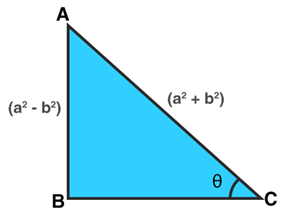Sin θ = (a2 – b2) / (a2 + b2)

AB = (a2 – b2)

AC = (a2 + b2)

By Pythagoras theorem:

BC = √[(a2 + b2)2 – (a2 – b2)2]

BC = √(4a2 b2)

or BC = 2ab

Find other T-rations using their definitions:

cos 𝜃 = base/hypotenuse = 2ab / (a2 + b2)

tan θ = perpendicular/base = (a2 – b2) / 2ab

cosec 𝜃 = 1/sin 𝜃 = (a2 + b2)/(a2 – b2)

sec θ = 1/cos 𝜃 = (a2 + b2)/2ab

cotθ = 1/tanθ = 2ab/(a2 – b2)

Question 7:
Solution:

Given: 15 cot A = 8

cotA = (8k)/(15k) = 1/tanA = AC/BC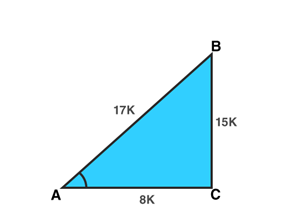Where k is any positive.

By Pythagoras theorem:

AB2 = BC2 + AC2

= (15k)2 + (8k)2

= 289k2

AB = 17k

Find other T-rations using their definitions:

sin A = perpendicular/ hypotenuse = (15k)/(17k) = 15/17

sec A = hypotenuse /base = 17/8

Question 8:
Solution:

Draw a triangle, ΔABC, Let ∠ACB = θ and ∠B = 90 degress

Sin A = perpendicular/ hypotenuse = 9/41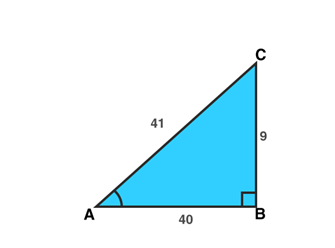By Pythagoras theorem:

AC2 = AB2 + BC2

AB2 = AC2 – BC2

= 412 – 92

= 1600

AB = 40

Find other T-ratios using their definitions:

cos A = base/ hypotenuse = 40/41

tan A = perpendicular /base = 9/40

Question 9:
Solution:

cos θ = 0.6 = (6k)/(10k) = AC/AB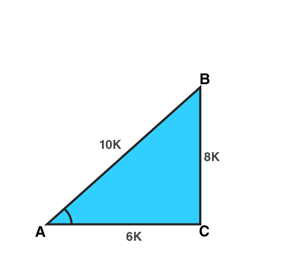Where k is any positive.

By Pythagoras theorem:

AB2 = BC2 + AC2

BC2 = AB2 – AC2

= (10k)2 + (6k)2

= 64k2

BC = 8k

Find other T-rations using their definitions:

sin θ = perpendicular/ hypotenuse = 8/10

tan θ = perpendicular/base = 8/6

Now,

LHS = 5sinθ – 3tanθ

= 5(8/10) – 3(8/6)

= 4 – 3(4/3)

= 4(3) – 3(4)

= 12 – 12

= 0

=RHS

Hence proved.

Question 10:
Solution:

cosec θ = 2

or 1/sinθ = 2

(cosecθ is reciprocal of sin θ)

sin θ = 1/2

which implies θ = 30 degrees.

Find the values of cos θ and cot θ at θ = 30 degrees.

cos 30^0 = √3/2 and cot 30^0 = √3

Now,

LHS = cot 0 + sin0/(1 +cos0)

= √3 + 1/2 / (1 + √3/2)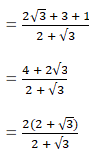=2

=RHS

Hence proved.

Question 11:
Solution:

Given: tan θ = 1/√7

tanθ = k/(k√7) = BC/AC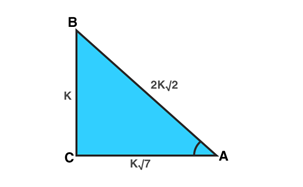Where k is any positive.

By Pythagoras theorem:

AB2 = BC2 + AC2

= k2 + 7k2

AB = 2k√2

Find cosec θ and sec θ using their definitions:

cosec θ = AB/BC = 2k√2/k = 2√2

secθ = AB/AC = 2√2/√7

Now,

LHS =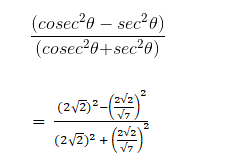= 48/64

=3/4

= RHS

Hence proved

Question 12.
Solution:

Given: tan θ = 20/21

tanθ = 20k/(21k)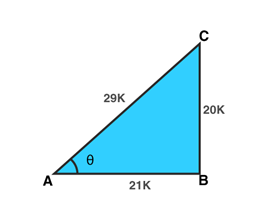Where k is any positive.

By Pythagoras theorem:

AC2 = AB2 + BC2

= 441k2 + 400k2

AC = 29k

Find sin θ and cos θ using their definitions:

sin θ = perpendicular/ hypotenuse = 20/29

cos 𝜃 = base/hypotenuse = 21/29

Now,

LHS =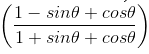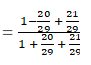=30/70

= 3/7

=RHS

Hence proved

Question 13:
Solution:

Given: secθ=5/4

sec θ = 5k/(4k) = 5/4 and cos θ = 4/5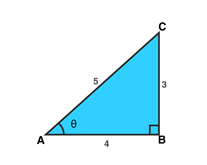Where k is any positive.

By Pythagoras theorem:

AC2 = AB2 + BC2

BC2 = AC2 – AB2

= 25k2 – 16k2

BC = 9k2 = 3k

Find sin θ, tan θ and cot θ using their definitions:

sin θ = perpendicular/ hypotenuse = 3/5

tan θ = perpendicular/base = 3/4

cotθ = 1/tanθ = 4/3

Now,

LHS =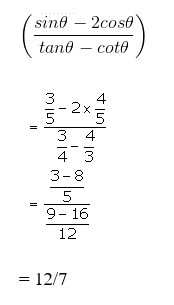=RHS

Question 14:
Solution:

Given: cotθ=3/4

or cotθ = 3k/4k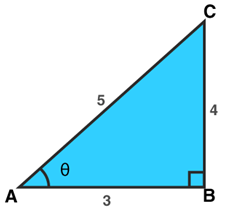Where k is any positive.

By Pythagoras theorem:

AC2 = AB2 + BC2

= 9k2 +16k2

= 25k2

AC = 5k

Find sec θ and cosec θ using their definitions:

sec θ = hypotenuse/base = 5/3

cosec 𝜃 = hypotenuse/perpendicular = 5/4

Now,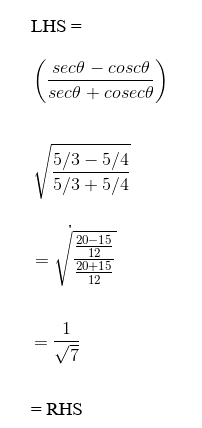Question 15:
Solution:

Given: sinθ=3/4

or sinθ=3k/4k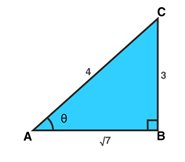Where k is any positive.

By Pythagoras theorem:

AC2 = AB2 + BC2

16k2 = AB2 + 9k2

AB = √7

Find sec θ and cot θ using their definitions:

sec θ = hypotenuse/base = 4/√7

cotθ = base/perpendicular = √7/3

Now,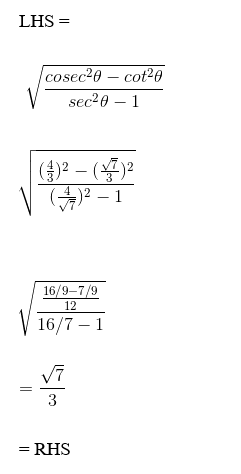Question 16:
Solution:

Given: sinθ = a/b

or sinθ= ak/bk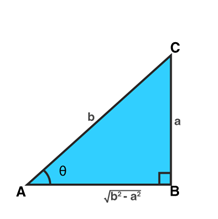Where k is any positive.

By Pythagoras theorem:

AC2 = AB2 + BC2

AB2 = AC2 – BC2

= b2 – a2

$\sqrt{b^{2}-a^{2}}$

Find sec θ and tan θ using their definitions:

sec θ = hypotenuse/base = b/√(b2 – a2)

tan θ = perpendicular/base = a/√(b2 – a2)

Now,

LHS = sec θ + tan θ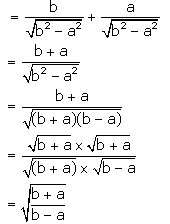= RHS

Question 17:
Solution:

Given: cosθ=3/5

cosθ = (3k)/(5k) = AC/AB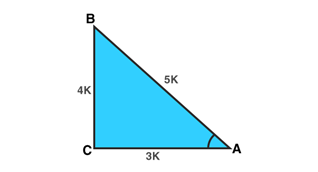Where k is any positive.

By Pythagoras theorem:

AB2 = BC2 + AC2

BC = 4k

Find sin θ, tan θ and cot θ using their definitions:

sin θ = perpendicular/ hypotenuse = 4/5

tan θ = perpendicular/base = 4/3

cot θ = 1/tan θ = 3/4

Now,

LHS =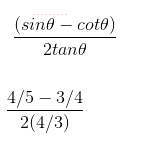= 3/160

= RHS

Question 18:
Solution:

Given: tan θ = 4/3

tanθ = (4k)/(3k) = BC/AC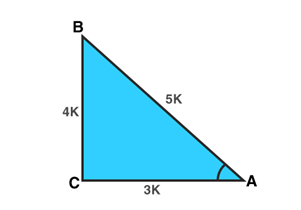Where k is any positive.

By Pythagoras theorem:

AB2 = BC2 + AC2

AB2 = 16k2 + 9k2

AB = 5k

Find sin θ and cos θ using their definitions:

sin θ = perpendicular/ hypotenuse = 4/5

cos 𝜃 = base/hypotenuse = 3/5

Now,

LHS = sin θ + cos θ

= 4/5 + 3/5

= 7/5

= RHS

Question 19:
Solution:

Given: tan θ = a/b

tanθ = (ak)/(bk) = BC/AB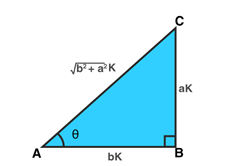Where k is any positive.

By Pythagoras theorem:

AC2 = AB2 + BC2

$AC = \sqrt{a^{2}+b^{2}}$

Find sin θ and cos θ using their definitions:

sin θ = perpendicular/ hypotenuse = a/(√(a2+b2)

cos 𝜃 = base/hypotenuse = b/(√(a2+b2)

Now,

LHS: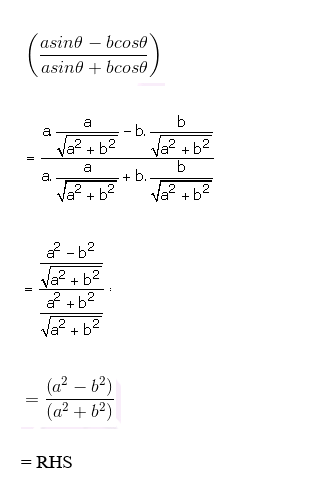Question 20:
Solution:

Given: 3 tan θ = 4

or tan θ = 4/3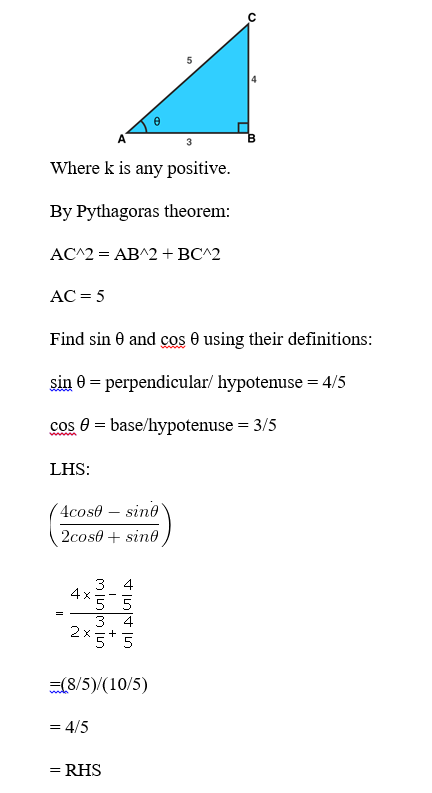Question 21:
Solution:

Given: 3 cot θ = 2

or cot θ = 2/3

Where k is any positive.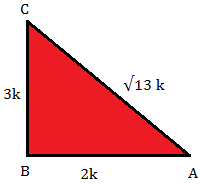By Pythagoras theorem:

AC2 = AB2 + BC2

AC2 = (2k)2 + (3k)2

AC2 = 4k2 + 9k2 = 13k2

AC = √13 k

Find sin θ and cos θ using their definitions:

sin θ = perpendicular/ hypotenuse = 3/√13

cos 𝜃 = base/hypotenuse =2/√13

Now,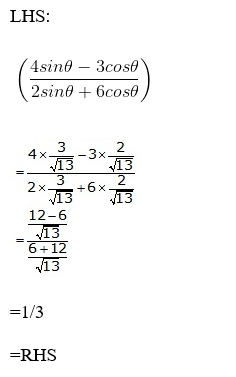Question 22:
Solution:

Given: 3cotθ=4

or cot θ = 4/3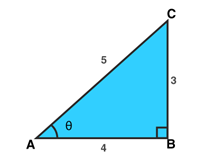Where k is any positive.

By Pythagoras theorem:

AC2 = AB2 + BC2

= 16 + 9

AC = 5 k

Find sin θ and cos θ using their definitions:

sin θ = perpendicular/ hypotenuse = 3/5

cos 𝜃 = base/hypotenuse = 4/5

Now,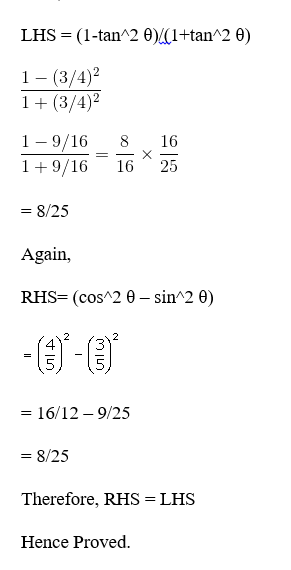Question 23:
Solution:

Given: sec θ = 17/8

or sec θ = 17k/8k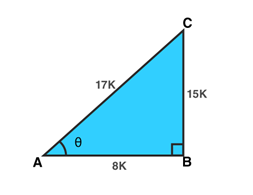Where k is any positive.

By Pythagoras theorem:

AC2 = AB2 + BC2

BC2 = AC2 – AB2

= 289k2 – 64k2

= 225 k2

BC = 15 k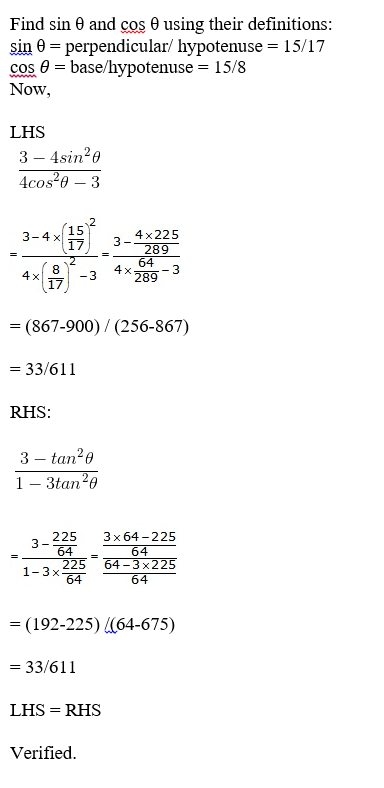Question 24:
Solution:

Draw a triangle using given instructions: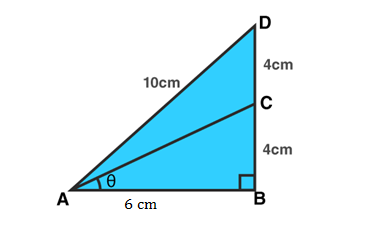From figure: Δ ABC and Δ ABD are right angled triangles

where AD = 10cm BC = CD = 4cm

BD = BC + CD = 8cm

By Pythagoras theorem:

(10)2 = (8)2 + AB2

100 = 64 + AB2

AB2 = 36 = (6)2

or AB = 6cm

Again,

AC2 = BC2 + AB2

AC2 = (4)2 + (6)2

AC2 = 16 + 36 = 52

or AC = √52 = 2√13 cm

(i) Find sin θ

sin θ = BC/AC = 4/2√13 = 2√13/13

(ii) Find cos θ

cosθ = AB/AC = 6/2√13 = 3/√13 = 3√13/13

Question 25:

Solution:

Draw a triangle using given instructions: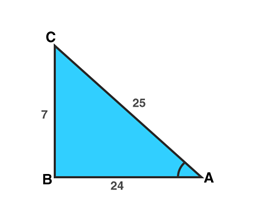From figure: Δ ABC is a right angled triangle

By Pythagoras theorem:

AC2 = BC2 + AB2

AC = 25

(i) Find sin A

sin A = BC/AC = 7/25

(ii) Find cos A

cos A = AB/AC = 24/25

(iii) sin C = AB/AC = 24/25

(iv) cosC = BC/AC = 7/25

Question 26:
Solution:

Draw a triangle using given instructions: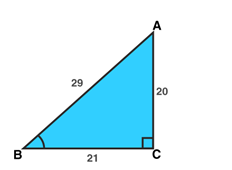From figure: Δ ABC is a right angled triangle

AB2 = AC2 + BC2

(29)2 = AC2 + (21)2

841 = AC2 + 441

AC2 = 400

or AC = 20

Find sin θ and cos θ:

sinθ = AC/AB = 20/29

cosθ = BC/AB = 21/29

Now:

LHS = cos2θ – sin2θ

= (21/29)2 – (20/29)2

= 41/841

= RHS

Hence proved.

Question 27:
Solution: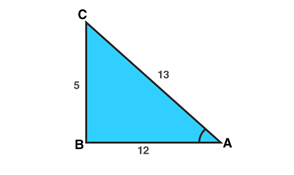From figure: Δ ABC is a right angled triangle

AC2 = BC2 + AB2

AC2 = (5)2 + (12)2

AC2 = 25 + 144

AC2 = 169 = (13)2

or AC = 13

Now from figure,

i. cos A = AB/AC = 12/13

ii. cosec A = AC/BC = 13/5

iii. cos C = BC/AC = 5/13

iv. cosec C = AC/AB = 13/12

Hence proved.

Question 28:
Solution:

Given: sinα = k/(2k) = BC/AB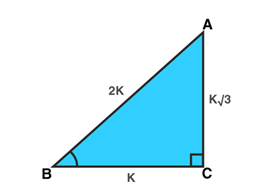Where k is any positive.

By Pythagoras theorem:

AB2 = BC2 + AC2

AC2 = AB2 – BC2

= (2k)2 – (k)2

= 3k2

or AC= k√3

Find cos α:

cos α = base/hypotenuse = √3/2

Now,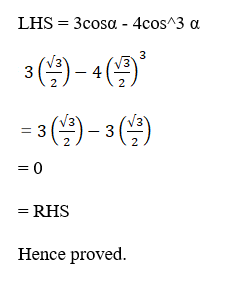Question 29:
Solution:

Given: tan A = BC/AB = k/(k√3)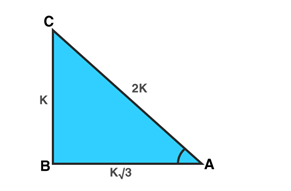Where k is any positive.

By Pythagoras theorem:

AC2 = BC2 + AB2

= (k)2 – (√3 k)2

= k2 + 3k2

= 4k2

or AC= 2k

Find sin A, cos A, sin C and cos C

sin A = BC/AC = k/(2k) = 1/2

cos A = AB/AC = (k√3)/(2k) = √3/2

sin C = AB/AC = (k√3)/(2k) = √3/2

cos C = BC/AC = k/(2k) = ½

(i) sinA cosC + cosA sinC = 1

LHS = sinA cosC + cosA sinC = (1/2)(1/2) + ( √3/2)( √3/2)

= 1/4 + 3/4

= 4/4

= 1

RHS = LHS

(ii) cosA cosC – sinA sinC = 0

LHS = cosA cosC – sinA sinC

= (1/2)(√3/2) – (1/2)(√3/2)

= (√3/4) – (√3/4)

= 0

=RHS

Question 30:
Solution:

Consider two right triangles XAY and WBZ such that sin A = sin B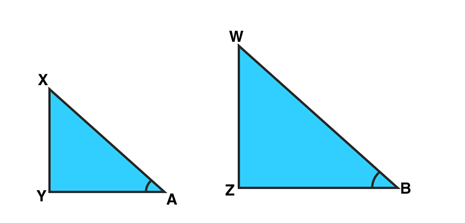To Prove: ∠A = ∠B

From figures:

sin A = XY/XA and sin B = WZ / WB

XY/XA = WZ / WB = k (say)

or XY/WZ = XA/ WB …(1)

sin A = sin B (Given)

We have,

XY = WZ k and XA = WB k …(2)

By Pythagoras: Apply on both the triangles

WB2 = WZ2 + BZ2

BZ2 = WB2 – WZ2

And,

XA2 = XY2 + AY2

AY2 = XA2 – XY2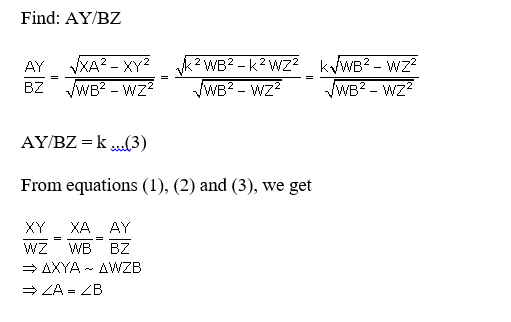Question 31:
Solution:

Consider ΔABC to be a right angled triangle.

angle C = 90 degree

tan A = BC/AC and

tan B = AC/BC

Given: tanA = tanB

So, BC/AC = AC/BC

BC2 = AC2

BC = AC

Which implies, ∠ A = ∠ B (using triangle opposite and equal angles property)

Question 32:
Solution:

Consider ΔABC to be a right angled triangle at B.

angle C = 90 degree

Given: tan A = 1 …(1)

tan A = 1 = BC/AB

AB = BC

Again, tan A = sin A/cos A

sin A = cos A …using (1)

By Pythagoras theorem:

AC2 = BC2 + AB2

AC2 = 2BC2

(AC/BC)2 = 2

Or AC/BC = √2

cosecA = √2

or sin A = 1/√2

and cosA = 1/√2

Now,

2 sinA cosA = 2(1/√2)( 1/√2)

= 2(1/2)

= 1

= RHS

Question 33:

Solution: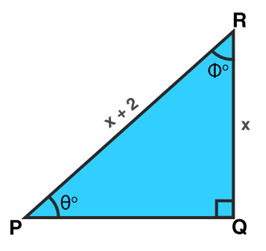Δ PQR is a right angled triangle.

By Pythagoras theorem:

PR2 = RQ2 + PQ2

(x + 2)2 = x2 + PQ2

PQ2 = 4 + 4x

or PQ = 2√(x+1)

Now,

cot ϕ = QR/PQ = x/2(√(x+1)

tan θ = QR/PQ = x/2(√(x+1)

(i) √(x+1) cot ϕ = √(x+1) {x/2(√(x+1)} = x/2

(ii) √(x^3 + x^ 2) tan θ = √(x^3 + x^ 2) {x/2(√(x+1)} = x2/2

(iii) cos θ = PQ/PR = 2(√(x+1) / (x+2)

Question 34: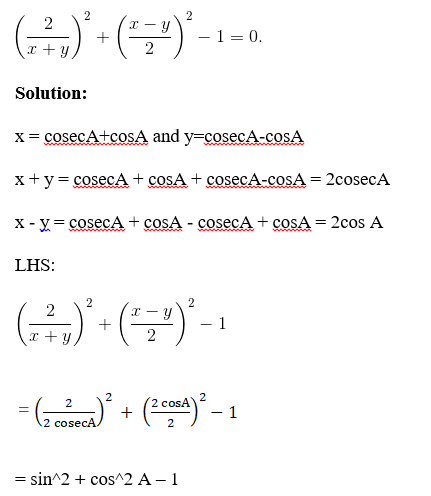(Using trig property: sin2 + cos2 A = 1)

= 1 – 1

= 0

Question 35: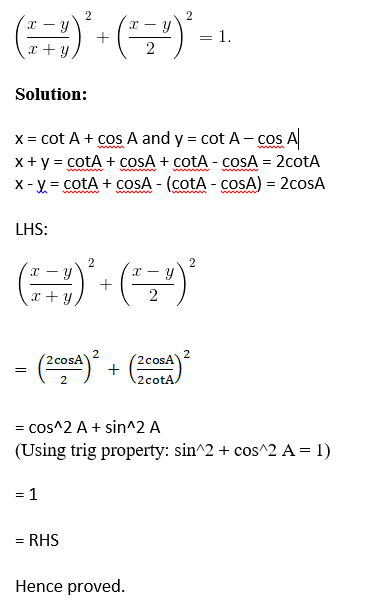## R S Aggarwal Solutions for Chapter 10 Trigonometry Ratios Topics

In this chapter students will study important concepts on Trigonometry Ratios as listed below:

• Trigonometry Ratios introduction
• Trigonometric ratios of an acute angle of a right triangle
• Reciprocal relations
• T-ratios of an angle
• Quotient relation of T-ratios
• Squared relation

### Key Features of RS Aggarwal Solutions for Class 10 Maths Chapter 10 Trigonometry Ratios

1. A simple and easily understandable approach is followed to solve all the questions.

2. These solutions will be useful for CBSE board exams, and other competitive exams.

3. The step-by-step problem-solving approach helps students to understand easily.

4. Easy for quick revision.

5. Detailed answers to all the questions to help students in their preparations.